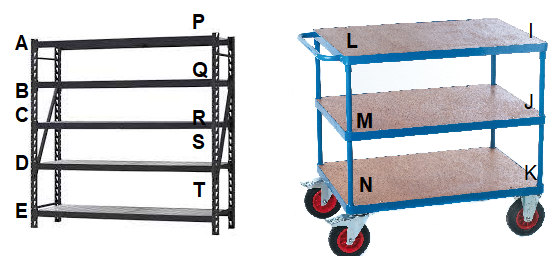# Math in Focus Grade 3 Chapter 17 Practice 3 Answer Key Perpendicular Lines

This handy Math in Focus Grade 3 Workbook Answer Key Chapter 17 Practice 3 Perpendicular Lines provides detailed solutions for the textbook questions.

## Math in Focus Grade 3 Chapter 17 Practice 3 Answer Key Perpendicular Lines

Guess if the lines are perpendicular. Use a ruler to check your guess.

Question 1.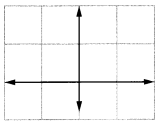The lines are perpendicular.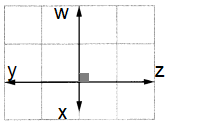Explanation:
If the intersection between the two line segment is at a right angle, then the two lines are perpendicular.
So, the angle between the wx and yz is 90 degrees.

Question 2.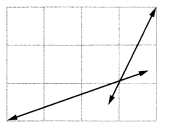The lines are not perpendicular.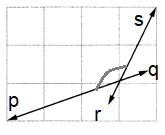Explanation:
If the intersection between the two line segment is at a right angle, then the two lines are perpendicular.
So, the angle between the pq and rs is greater then 90 degrees.

Question 3.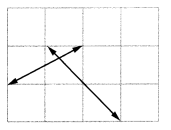The lines are not perpendicular.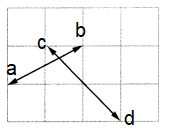Explanation:
If the intersection between the two line segment is at a right angle, then the two lines are perpendicular.
So, the angle between the ab and cd is greater then 90 degrees.

Question 4.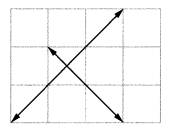The lines are perpendicular and the angle between the ij and kl is 90 degrees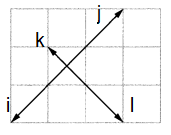If the intersection between the two line segment is at a right angle, then the two lines are perpendicular,

Question 5.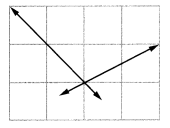The lines are not perpendicular.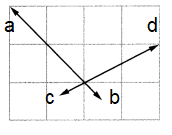Explanation:
If the intersection between the two line segment is at a right angle, then the two lines are perpendicular.
So, the angle between the ab and cd is greater then 90 degrees.

Question 6.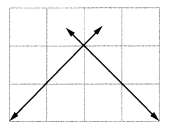The lines are perpendicular.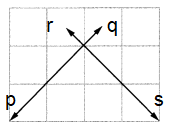Explanation:
If the intersection between the two line segment is at a right angle, then the two lines are perpendicular.
So, the angle between the pq and rs is 90 degrees.

Check (✓) the box if the lines are perpendicular.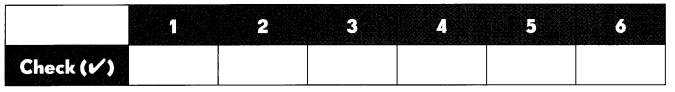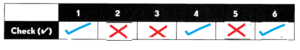Explanation:
From the above figures we can come to conclusion that figures 1, 4 and 6 are parallel lines and
figures 2,3 and 5 are not parallel lines.

Use a ruler to check for perpendicular lines. Use a colored pencil to trace a pair of perpendicular lines in each figure.

Question 7.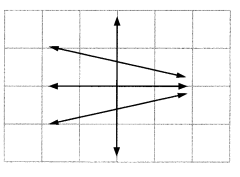The lines are perpendicular.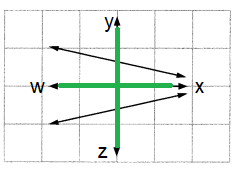Explanation:
If the intersection between the two line segment is at a right angle, then the two lines are perpendicular.
So, the angle between the wx and yz is 90 degrees.

Question 8.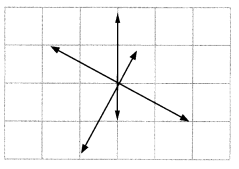The lines are perpendicular.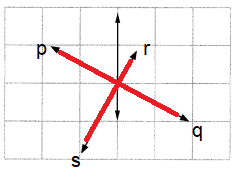Explanation:
If the intersection between the two line segment is at a right angle, then the two lines are perpendicular.
So, the angle between the pq and rs is 90 degrees.

Question 9.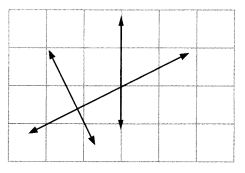The lines are perpendicular.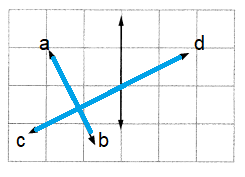Explanation:
If the intersection between the two line segment is at a right angle, then the two lines are perpendicular.
So, the angle between the pq and rs is 90 degrees.

Question 10.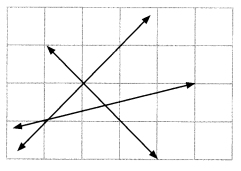The lines are perpendicular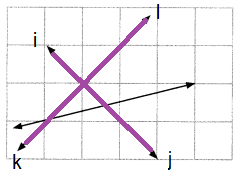Explanation:
If the intersection between the two line segment is at a right angle, then the two lines are perpendicular.
So, the angle between the ij and kl is 90 degrees.

Circle the letters that have perpendicular line segments.

Question 11.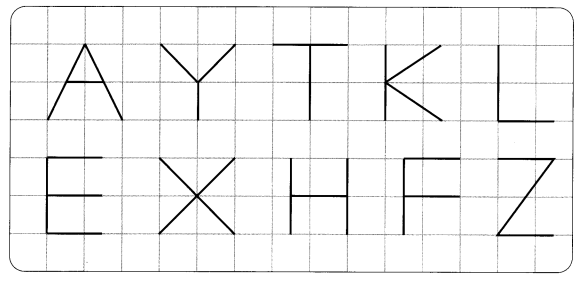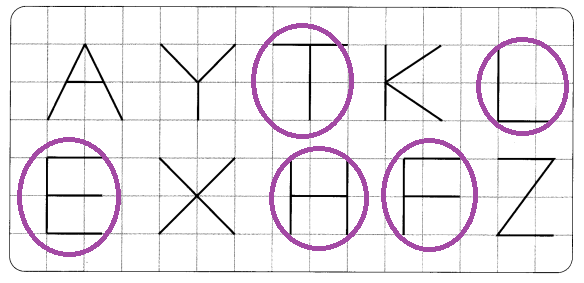Explanation:
If the intersection between the two line segment is at a right angle, then the two lines are perpendicular.
So, the letters T,L,E,H,F are perpendicular line segmests.

Name all the pairs of perpendicular line segments in each figure.

Example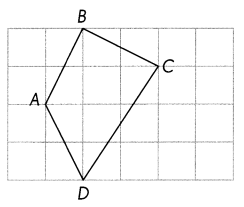Segments AB and BC

Question 12.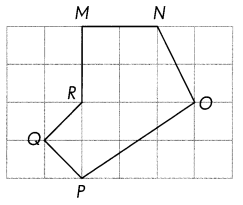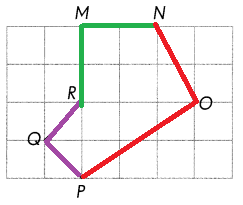The pairs of perpendicular line segments
Segments RQ and QP
Segments OP and ON
Segments MN and MR
Explanation:
If the intersection between the two line segment is at a right angle, then the two lines are perpendicular.
The colors of lines indicate different pairs of line segments.

Question 13.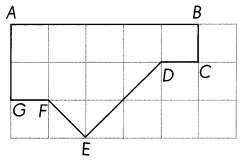The pairs of perpendicular line segments are:
Segments AB and AG
Segments AB and BC
Segments FE and DE
Explanation:
If the intersection between the two line segment is at a right angle, then the two lines are perpendicular.
The colors of lines indicate different pairs of line segments.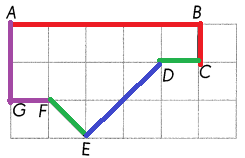Question 14.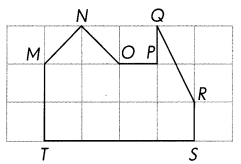The pairs of perpendicular line segments are:
Segments MT and TS
Segments RS and TS
Segments OP and PQ
Explanation:
If the intersection between the two line segment is at a right angle, then the two lines are perpendicular.
The colors of lines indicate different pairs of line segments.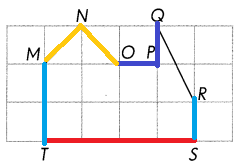Identify and name a pair of perpendicular line segments on each object.

Question 15.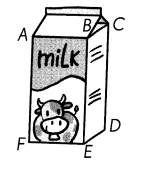A pair of perpendicular line segments on each object are,
AF and FE
BE and ED
CD and DE are a pair of perpendicular line segments.
Explanation:
If the intersection between the two line segment is at a right angle, then the two lines are perpendicular.

Question 16.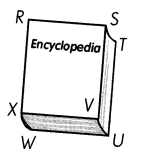A pair of perpendicular line segments on Encyclopedia are,
RS and RX
SV and VX
SV and VU are a pair of perpendicular line segments.
Explanation:
If the intersection between the two line segment is at a right angle, then the two lines are perpendicular.

Carry out this activity.

Question 17.
Think of two objects that have perpendicular line segments. Use the Internet to find pictures of these objects. Print and paste them below. Use a colored pencil to trace a pair of perpendicular line segments on each object.